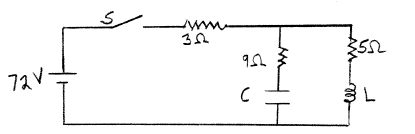Physics Practice Problems LRC Circuits Practice Problems Solution: Consider the circuit shown in the sketch. C = 5.0 ...

# Solution: Consider the circuit shown in the sketch. C = 5.0 x 10-6 F and L = 0.30 H. Initially the switch S is open, there are no currents, and there is no charge on the capacitor. Then the switch is closed.  After the switch has been closed a long time, what is the voltage across the 3 Ω resistor?

###### Problem

Consider the circuit shown in the sketch. C = 5.0 x 10-6 F and L = 0.30 H. Initially the switch S is open, there are no currents, and there is no charge on the capacitor. Then the switch is closed.  After the switch has been closed a long time, what is the voltage across the 3 Ω resistor?